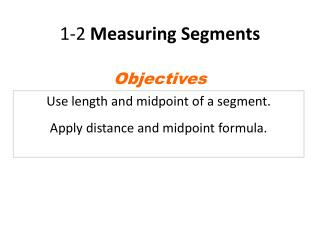DownloadDownload Presentation1-2 Measuring Segments

# 1-2 Measuring Segments

Télécharger la présentation## 1-2 Measuring Segments

- - - - - - - - - - - - - - - - - - - - - - - - - - - E N D - - - - - - - - - - - - - - - - - - - - - - - - - - -
##### Presentation Transcript

1. 1-2 Measuring Segments Objectives Use length and midpoint of a segment. Apply distance and midpoint formula.

2. Vocabulary coordinatemidpoint distancebisect lengthsegmentbisectorcongruent segments

3. A point corresponds to one and only one number (or coordinate) on a number line.

4. A B AB = |a – b| or |b - a| a b Distance (length): the absolute value of the difference of the coordinates.

5. Example 1: Finding the Length of a Segment Find each length. A. BC B. AC BC = |1 – 3| AC = |–2 – 3| = |– 2| = |– 5| = 2 = 5

6. Point B is betweentwo points A and Cif and only if all three points are collinear and AB + BC = AC. A

7. bisect: cut in half; divide into 2 congruent parts. midpoint: the point that bisects, or divides, the segment into two congruent segments

8. 4x + 6 = 7x - 9 +9 +9 4x + 15 = 7x -4x -4x 15 = 3x 3 5 = x

9. It’s Mr. Jam-is-on Time!

10. Recap! 1. M is between N and O. MO = 15, and MN = 7.6. Find NO. 2.S is the midpoint of TV, TS = 4x – 7, and SV = 5x – 15. Find TS, SV, and TV. 3. LH bisects GK at M. GM =2x + 6, and GK = 24.Find x.

11. 1. M is between N and O. MO = 15, and MN = 7.6. Find NO.

12. 2. S is the midpoint of TV, TS = 4x – 7, and SV = 5x – 15. Find TS, SV, and TV.

13. 3.LH bisects GK at M. GM =2x + 6, and GK = 24.Find x.

14. 1-6 Midpoint and Distance in the Coordinate Plane

15. Vocabulary • Coordinate plane: a plane that is divided into four regions by a horizontal line called the x-axis and a vertical line called the y-axis. y-axis The location, or coordinates, of a point is given by an ordered pair (x, y). II I x-axis III IV

16. Midpoint Formula The midpoint M of a AB with endpoints A(x1, y1) and B(x2, y2) is found by

17. Example Find the midpoint of GH with endpoints G(1, 2) and H(7, 6).

18. Example M(3, -1) is the midpoint of CD and C has coordinates (1, 4). Find the coordinates of D.

19. Distance Formula The distance d between points A(x1, y1) and B(x2, y2) is

20. Example Use the Distance Formula to find the distance between A(1, 2) and B(7, 6).

21. Pythagorean Theorem In a right triangle, a2 + b2 = c2 c is the hypotenuse (longest side, opposite the right angle) a and b are the legs (shorter sides that form the right angle)

22. Example Use the Pythagorean Theorem to find the distance between J(2, 1) and K(7 ,7).